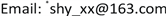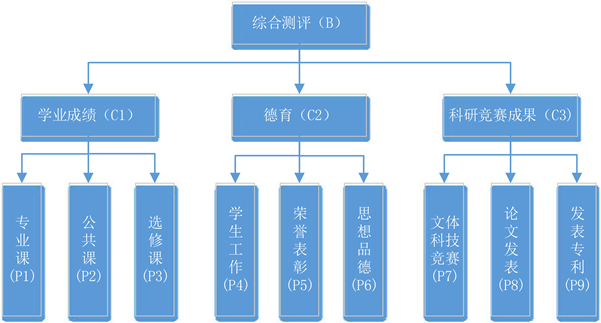﻿ 基于改进层次分析法的大学生综合测评研究 Research on Comprehensive Evaluation of College Students Based on Improved Analytic Hierarchy Process

Creative Education Studies
Vol. 07  No. 03 ( 2019 ), Article ID: 30712 , 7 pages
10.12677/CES.2019.73048

Research on Comprehensive Evaluation of College Students Based on Improved Analytic Hierarchy Process

Liming Yan1, Haiyi Sun1*, Ning Li2

1College of Science, Shenyang Jianzhu University, Shenyang Liaoning

2College of Sciences, Northeastern University, Shenyang LiaoningReceived: May 20th, 2019; accepted: June 4th, 2019; published: June 11th, 2019ABSTRACT

An analytic hierarchy process (AHP) model for interval estimation of weight vectors is established by using constrained cones and mathematical programming. The model regards the preference information of decision-makers as a constraint, which can well reflect the uncertainty in the decision-making process and the preference degree of decision-makers. Then, the AHP indicators were screened in order to solve the unavoidable deviations and errors of decision makers when using analytic hierarchy process, and the inconsistency of judgment matrix caused by them. We can’t regard index weight and judgment matrix as a definite functional dependence. We should deal with this correlation from an uncertain perspective. Therefore, when screening indicators, interval estimation is used to eliminate weak weight indicators, and a complete, objective, concise and easy-to-measure index system is constructed scientifically and reasonably. Finally, the model of comprehensive quality evaluation of college students based on interval estimation of analytic hierarchy process (AHP) is established, applying the comprehensive evaluation model and building the comprehensive quality evaluation system of students based on the network platform.

Keywords:Constraint Cone, Interval Estimation, Analytic Hierarchy Process (AHP), Index Screening, Comprehensive Evaluation

1沈阳建筑大学理学院，辽宁 沈阳

2东北大学理学院，辽宁 沈阳1. 引言

1.1. 研究意义

1.2. 国内外研究现状

2. 改进层次分析法

2.1. 计算权重向量

${B}_{n}={\left({b}_{ij}\right)}_{n×n}$ (1)

${\lambda }_{\mathrm{max}}$ 为判断矩阵 ${B}_{n}$ 的最大特征值，则权重矩阵为：

$\omega ={\left({\omega }_{1},{\omega }_{2},\cdots ,{\omega }_{n}\right)}^{T}$ (2)

$\left({b}_{i1},{b}_{i2},\cdots ,{b}_{in}\right)×\omega \le {\lambda }_{\mathrm{max}}{\omega }_{i}\left(i=1,2,\cdots ,n\right)$ (3)

${B}_{n}\omega \le {\lambda }_{\mathrm{max}}\omega$。令

${\stackrel{¯}{B}}_{n}={B}_{n}-{\lambda }_{\mathrm{max}}{E}_{n}$ (4)

${\stackrel{¯}{B}}_{n}\omega \le 0\left(\omega \ge 0\right)$ (5)

$\begin{array}{l}\mathrm{max}\left(或\mathrm{min}\right).{\omega }_{i}\\ s.t.\left\{\begin{array}{c}{\stackrel{¯}{B}}_{n}\omega \le 0\\ \omega ={\left({\omega }_{1},{\omega }_{2},\cdots ,{\omega }_{n}\right)}^{T}\\ {\omega }_{1}+{\omega }_{2}+\cdots +{\omega }_{n}=1\end{array}\end{array}$ (6)

2.2. 弱权重指标的剔除

${\Delta }_{\mathrm{max}}=\underset{1\le i\le n}{\mathrm{max}}\left({\omega }_{iU}-{\omega }_{iL}\right)$ (7)

${\omega }_{iU}\le {\Delta }_{\mathrm{max}}$ (8)

3. 建立高校学生综合测评系统

3.1. 建立层次结构模型

3.2. 构造判断矩阵Figure 1. Comprehensive evaluation hierarchy

3.3. 一致性检验和层次排序

$CI=\frac{{\lambda }_{\mathrm{max}}-n}{n-1}$ (9)

$CR=\frac{CI}{RI}$ (10)

3.4. 基于AHP排序结果构建综合测评系统

$\begin{array}{l}B=C1×{\omega }_{1}+C2×{\omega }_{2}+C2×{\omega }_{3}\\ C1=P1×{\omega }_{11}+P2×{\omega }_{12}+P3×{\omega }_{13}\\ C2=P4×{\omega }_{21}+P5×{\omega }_{22}+P6×{\omega }_{23}\\ C3=P7×{\omega }_{31}+P8×{\omega }_{32}+P9×{\omega }_{33}\end{array}$ (11)

3.5. 算例

3.6. 搭建学生综合测评平台

4. 总结与展望

Research on Comprehensive Evaluation of College Students Based on Improved Analytic Hierarchy Process[J]. 创新教育研究, 2019, 07(03): 275-281. https://doi.org/10.12677/CES.2019.73048

1. 1. 高杰, 孙林岩, 何进, 等. 层次分析的区间估计[J]. 系统工程理论与实践, 2004, 24(3): 103-106.

2. 2. 程瑶. 基于改进层次分析法的高校学生综合素质测评模型研究[D]: [硕士学位论文]. 马鞍山: 安徽工业大学, 2018.

3. 3. 胡晓天, 沈桂芳, 周园园, 等. 基于层次分析法与信息熵的大学生综合素质评估模型构建研究[J]. 赤峰学院学报(自然科学版), 2017, 33(1): 219-221.

4. 4. 李春霞, 刘琪. 层次分析法在学生综合素质评价中的应用[J]. 科技视界, 2017(9): 61-62.

5. 5. 高杰, 孙林岩, 李满圆. 区间估计: AHP指标筛选的一种方法[J]. 系统工程理论与实践, 2005, 25(10): 73-77.

6. 6. T.L. Saaty. 层次分析法[M]. 许树柏, 译. 北京: 煤炭工业出版社, 1988.

7. 7. 岳志强. 基于层次分析法的高校学生综合测评量化平台研究[J]. 福州大学学报(哲学社会科学版), 2015, 29(6): 94-98.

8. 8. 徐慧, 罗超, 刘志刚. 层次分析法评价指标筛选方法探讨[J]. 中国海上油气, 2007, 19(6): 415-418.

9. NOTES

*通讯作者。